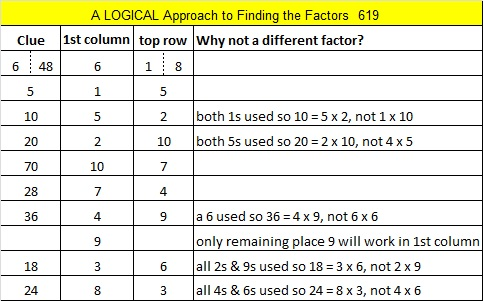# 619 and Level 4

619  is the 12th strobogrammatic number because it looks like the same number when it is turned upside down. The first 4 strobogrammatic prime numbers are 11, 101, 181, and 619.Print the puzzles or type the solution on this excel file: 10 Factors 2015-09-14

—————————————————————————————————

• 619 is a prime number.
• Prime factorization: 619 is prime.
• The exponent of prime number 619 is 1. Adding 1 to that exponent we get (1 + 1) = 2. Therefore 619 has exactly 2 factors.
• Factors of 619: 1, 619
• Factor pairs: 619 = 1 x 619
• 619 has no square factors that allow its square root to be simplified. √619 ≈ 24.87971.How do we know that 619 is a prime number? If 619 were not a prime number, then it would be divisible by at least one prime number less than or equal to √619 ≈ 24.9. Since 619 cannot be divided evenly by 2, 3, 5, 7, 11, 13, 17, 19, or 23, we know that 619 is a prime number.

—————————————————————————————————This site uses Akismet to reduce spam. Learn how your comment data is processed.